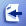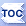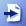# Math Parser for PHP Test Drive

You can use the html form below to try Math Parser for PHP functionality. Math parser parses  and evaluates mathematical expressions given as strings without using the PHP eval() function for security reasons. The syntax of the math expression is therefore is not PHP syntax. It is rather a more natural syntax we are used to see in Excel and other expression calculators.

Here we showcase the default functionality with two variables x, y and predefined functions. Math parser allows you to define your own variables and functions and you are not limited to this example set of functions shown in this example.

The math formula you input as an expression will be parsed by the PHP math parser library and evaluated for the X and Y values you provide below.

For example, enter X+Y as the expression, give 5 for X and 2 for Y. Result will be 7.

Current PHP version: 7.2.34

 Expression: X: Y:

Result: Please enter an expression that contains X, Y and mathematical functions and press the calculate button.

Predefined functions are:

SQR: Square function which can be used as SQR(X)

SIN: Sinus function which can be used as SIN(X), X is a real-type expression. Sin returns the sine of the angle X in radians.

COS: Cosinus function which can be used as COS(X), X is a real-type expression. COS returns the cosine of the angle X in radians.

ATAN: ArcTangent function which can be used as ATAN(X) Returns the arctangent of a number as a numeric value between -PI/2 and PI/2 radians.

SINH: Sinus Hyperbolic function which can be used as SINH(X)

COSH: Cosinus Hyperbolic function which can be used as COSH(X)

COTAN: which can be used as COTAN(X)

TAN: which can be used as TAN(X)

EXP: which can be used as EXP(X)

LN: natural log, which can be used as LN(X)

LOG: 10 based log, which can be used as LOG(X)

SQRT: which can be used as SQRT(X)

ABS: absolute value, which can be used as ABS(X)

SIGN: SIGN(X) returns -1 if X<0; +1 if X>0, 0 if X=0; it can be used as SQR(X)

TRUNC: Discards the fractional part of a number. e.g. TRUNC(-3.2) is -3, TRUNC(3.2) is 3.

CEIL: CEIL(-3.2) = 3, CEIL(3.2) = 4

FLOOR: FLOOR(-3.2) = -4, FLOOR(3.2) = 3

VAL: VAL("3.1") = 3.1 Returns the floating point numeric value of the string argument.

Predefined functions that take two parameters are:

POW: The Power function raises Base to any power. For fractional exponents or exponents greater than MaxInt, Base must be greater than 0.

LOGN: The LogN function returns the log base N of X. Example: LOGN(10, 100) = 2

MIN: MIN(2, 3) is 2.

MAX: MAX(2, 3) is 3.

IF: The IF(b, case1, case2) function provides branching capability. If b is not 0, then it returns case1, else it returns case2. Behavior is similar to PHP's: return b ? case1 : case2;
If b==0 then case1 will not be Evaluated, and vice versa. Example: IF(HEIGHT, 3/HEIGHT, 3) will make sure 3/HEIGHT does not cause division by zero.

Predefined functions that take no parameters are: RND: RND() function generates a random number (double value) between 0 and 1.

STR: STR(123) function returns the string representation of the passed value: "123".

SUBSTR: SUBSTR("Hello", 1,3) function returns the substring just like PHP substring function. The first parameter is the string, the second parameter is which index (0 based) to start copying, and the last parameter is the number of characters to copy. For example, SUBSTR("Hello", 1,3) returns "ell".

STRLEN: STRLEN("abc") function returns the length of the string parameter. For example, for "abc" it returns 3.

CONCAT: CONCAT("abc","def",...) function returns the concatanated strings: "abcdef". There is no preset limit on the number of parameters.

TRIM: TRIM(" abc ") function returns the trimmed version of the string parameter: " abc " -> "abc".

SUM: SUM(2,3,5,...) functions returns the sum of it's arguments. There is no preset limit on the number of parameters.

User functions can be added using CreateFunc method. Functions and Variables can be deleted using DeleteVar, DeleteFunc, DeleteAllVars, DeleteAllFuncs methods.

webmaster@gobestcode.com